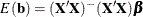# The REG Procedure

### Models of Less Than Full Rank

If the model is not full rank, there are an infinite number of least squares solutions for the estimates. PROC REG chooses a nonzero solution for all variables that are linearly independent of previous variables and a zero solution for other variables. This solution corresponds to using a generalized inverse in the normal equations, and the expected values of the estimates are the Hermite normal form ofmultiplied by the true parameters:Degrees of freedom for the zeroed estimates are reported as zero. The hypotheses that are not testable have t tests reported as missing. The message that the model is not full rank includes a display of the relations that exist in the matrix.

The following statements use the fitness data from Example 97.2. The variable `Dif`=`RunPulse``RestPulse` is created. When this variable is included in the model along with `RunPulse` and `RestPulse`, there is a linear dependency (or exact collinearity) between the independent variables. Figure 97.36 shows how this problem is diagnosed.

```data fit2;
set fitness;
Dif=RunPulse-RestPulse;
run;
proc reg data=fit2;
model Oxygen=RunTime Age Weight RunPulse MaxPulse RestPulse Dif;
run;
```

Figure 97.36: Model That Is Not Full Rank: REG Procedure

The REG Procedure
Model: MODEL1
Dependent Variable: Oxygen

Analysis of Variance
Source DF Sum of
Squares
Mean
Square
F Value Pr > F
Model 6 722.54361 120.42393 22.43 <.0001
Error 24 128.83794 5.36825
Corrected Total 30 851.38154

 Root MSE R-Square 2.31695 0.8487 47.3758 0.8108 4.89057

Parameter Estimates
Variable DF Parameter
Estimate
Standard
Error
t Value Pr > |t|
Intercept 1 102.93448 12.40326 8.30 <.0001
RunTime 1 -2.62865 0.38456 -6.84 <.0001
Age 1 -0.22697 0.09984 -2.27 0.0322
Weight 1 -0.07418 0.05459 -1.36 0.1869
RunPulse B -0.36963 0.11985 -3.08 0.0051
MaxPulse 1 0.30322 0.13650 2.22 0.0360
RestPulse B -0.02153 0.06605 -0.33 0.7473
Dif 0 0 . . .

PROC REG produces a message informing you that the model is less than full rank. Parameters with DF=0 are not estimated, and parameters with DF=B are biased. In addition, the form of the linear dependency among the regressors is displayed.